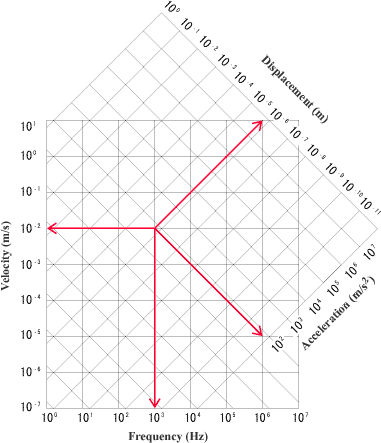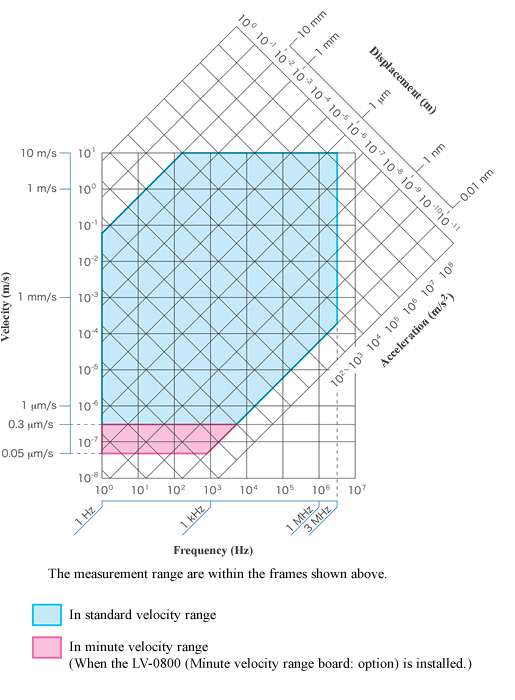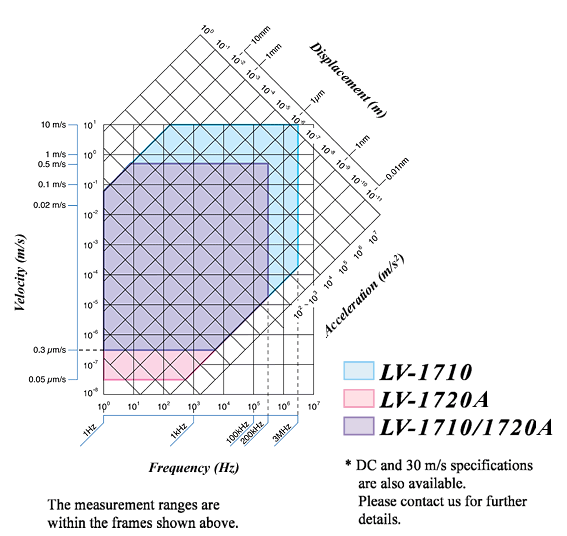Laser Doppler Vibrometer Measurement Range

Measuring range is defined in the scale chart represented by four parameters of frequency, velocity, acceleration and displacement. The Laser Doppler Vibrometer detects vibration velocity of an object. Therefore, the measurable range area is basically represented by two of parameter of velocity expressed in Y-axis and frequency expressed in X-axis. Furthermore, with the velocity and the frequency, acceleration can be calculated by differential and displacement can be obtained by integration. Suppose that values of velocity and frequency are given, acceleration value, which is differential value, is obtained by following the line downward to the right from an intersection point of velocity and frequency in the scale chart. And it also reaches to the displacement value, which is the integral value, by following the line upward to the right. An FFT analyzer can easily calculate acceleration and displacement by using its calculation function. Be noted, measuring range of velocity is expressed in half of amplitude since the vibration is a reciprocating motion.(Example)

This is an example of measurement of vibration on an object using the LV-series laser vibrometer.

Velocity : 10mm/s (converted from voltage)
Frequency : 1 kHz (calculated by FFT analyzer)

These two parameters are within the measurable range area rather than measuring range.

Acceleration value can be obtained by conversion and is be approx. 63m/s². (approx. 6.4G) (Acceleration velocity x 2 πf)

And, displacement is approx. 1.6 μm. (Displacement velocity/2 πf)

Both value is expressed in half of amplitude.

LV-1800 series Measurement RangeLV-1700 series Measurement Range